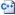# XWV

Stub

XWV is the sound format used by Valve's Xbox 360 games. It is similar to the WAV format and uses the big-endian byte order. The format is defined in the SDK in`public\xwvfile.h`.

```#define XWV_ID		(('X'<<24)|('W'<<16)|('V'<<8)|(' '<<0))
#define XWV_VERSION 4

enum xwvSampleRate_t
{
XWV_RATE_11025 = 0,
XWV_RATE_22050 = 1,
XWV_RATE_44100 = 2,
};

enum xwvFormat_t
{
XWV_FORMAT_PCM = 0,
XWV_FORMAT_XMA = 1,
};

// generated in big-endian
{
unsigned int	id;
unsigned int	version;
unsigned int	staticDataSize;		// follows header
unsigned int	dataOffset;		// start of samples, possibly sector aligned
unsigned int	dataSize;		// length of samples in bytes
unsigned int	numDecodedSamples;	// for duration calcs
int		loopStart;		// -1 = no loop, offset of loop in samples
unsigned short	loopBlock;		// the xma block where the loop starts